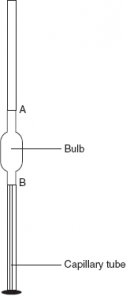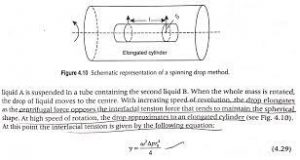# Drop weight and drop count method and Spinning Drop Method and MCQs for GPAT, NIPER, Pharmacist and Drug Inspector exam

## Drop weight and drop count method and Spinning Drop Method and MCQs for GPAT, NIPER, Pharmacist and Drug Inspector exam

Drop Weight and Drop Count Method:

Principle: If a liquid is allowed to fall slowly through a capillary tube, the liquid first forms a drop at the tip of the capillary tube, which gradually increases in size and finally detaches when the weight of the drop (W) equals the total surface tension at the circumference of the tube (2πr). Expressing mathematically,

W = 2πrᵧ

On the basis of the above principle, the surface tension of a liquid can be determined by either (1) the drop weight method or (2) the drop count method using a stalagmometer.

The stalagmometer or drop pipette consists of a glass tube with a bulb blown approximately in the middle of the tube and a circular flattened end. There are two markings A and B on the tube, one above the bulb and the other below it. There is a capillary bore at the tip of the stalagmometer just above the flattened end.Fig 1 – Schematic representation of stalagmometer (taken from Liquid Mr. Education)

A total of 20–30 drops are collected into a clean vessel and the weight of one drop of the liquid is determined (w1). After this, the liquid with the known surface tension (water) is taken up in the pipette and the weight of its one drop (w2) is obtained.

The relative surface tension of the liquid is then determined by the following equation:

Surface tension of the liquid / Surface tension of water = (w1/2πr)/(w2/2πr) = w1/w2

The drop count method is similar to the drop weight method except that the number of drops of the liquid formed when the liquid level falls from mark A to B is counted instead of weighing.

We know that:

ᵞ = weight of one drop of liquid / 2πr = mg /2πrn

where m is the mass of one drop, g the gravitational force and n the number of drops.

Or

ᵞ = VǷg / 2πrn

where v is the volume and Ƿ the density.

To determine the relative surface tension of a liquid, the number of drops of the liquid (n1) as well as of water (n2) formed for the same volume is determined. The relative surface tension of the liquid is given by

Surface tension of the liquid / Surface tension of water = (VǷ1g / 2πrn1) / (VǷ2g / 2πrn2)

= Ƿ1 n2 / Ƿ2 n1

1. The method is easily adapted for both surface and interfacial tensions determinations.
2. The drop should be formed slowly.
3. The tip must be completely wetted by the liquid, and the drop should not climb up the outside of the tube.
4. Correction factor is required as not all the drops leave the tip on detachment.
5. Correction factor depends on the radius of the tip and the drop volume.

Spinning Drop Method:

This method is particularly useful for measuring very low interfacial tensions (< 10–1 mN m–1), which are especially important in the formation of microemulsions. A drop of the less-dense liquid A is suspended in a tube containing the second liquid B. When the whole mass is rotated, the drop of liquid moves to the center. With increasing speed of revolution, the drop elongates as the centrifugal force opposes the interfacial tension force that tends to maintain the spherical shape. At high speed of rotation, the drop approximates to an elongated cylinder.

At this point the interfacial tension is given by the following equation:

ᵞ = ω2∆pro4 / 4Fig 2 – Schematic representation of a spinning drop method (taken from Gaurav Kumar Jain – Theory and Practice of Physical Pharmacy)

Multiple choice questions (MCQs)
1. Drop Weight and Drop Count Method is determined using
a) Pycnometer

b)Hydrometer

c)Stalagmometer

d)All of the above

2. If a liquid is allowed to fall slowly through a capillary tube, the liquid first forms a drop at the tip of the capillary tube, which gradually increases in size and finally detaches when the weight of the drop (W) equals the total surface tension at the circumference of the tube (2πr). This principle is involved in which of the following methods?
a) The drop weight method

b)The drop count method

c)Spinning Drop Method

d) a) and b)

3. Surface tension is a/an
a) Capacity factor
b) Extensive property
c) Intensive property
d) Tolerance factor

4. When the angle of contact is below liquid and solid is 90º then
b) fc,< fa
c) fc= fa
d) fc>> fa

5. This method is particularly useful for measuring very low interfacial tensions (< 10–1 mN m–1), which are especially important in the formation of microemulsions.

a) The drop weight method

b)The drop count method

c)Spinning Drop Method

d) a) and b)

6. The apparatus used for the determination of surface tension of a liquid is ____________
a) Oedometer
b) Stalagmometer
c) Consolidometer
d) None of the mentioned

7. Stalagmometer is cleaned to remove grease with the help of __________.
a) Chromic acid
b) Sulphuric acid
c) Distilled water
d) Tartaric acid

8. When there are no external forces, the shape of a liquid drop is determined by
a) Surface Tension of the liquid
(b) The density of the liquid
(c) The viscosity of the liquid
(d) The temperature of air only

9. At the critical temperature, the surface tension of the liquid
a) Is zero
(b) Is infinity
(c) Is the same as that at the other temperature
(d) Cannot be determined

10. The surface tension of a liquid can be determined by
a) The drop weight method

b)The drop count method

c)Spinning Drop Method

d)All of the above

11. How many drops are collected into a clean vessel in case of drop weight method?
a) 1-10

b)10-20

c)20-30

d)30-40

12. The relative surface tension of the liquid by drop weight method is determined by which of the following equation?
a) Surface tension of the liquid / Surface tension of water = (w2/2πr)/(w2/2πr) = w1/w2

b)Surface tension of the liquid / Surface tension of water = (w1/2πr)/(w2/2πr) = w1/w2

c)Surface tension of the liquid / Surface tension of water = (w1/2πr)/(w1/2πr) = w1/w2

d)Surface tension of the liquid / Surface tension of water = (w1/2πr)/(w2/2πr) = w1/w1

13. The relative surface tension of the liquid by drop count method is given by which of the following equation?
a) Surface tension of the liquid / Surface tension of water = (VǷ1g / 2πrn1) / (VǷ2g / 2πrn2)

b)Surface tension of the liquid / Surface tension of water = Ƿ1 n2 / Ƿ2 n1

c)Both of these

d)only a)

14. Antoniff’s rule is applicable to
a) Highly polar liquid against water
b) Non polar liquid against water
c) Polar liquids against any other immiscible liquids
d) Slightly polar liquids against water

15. The interfacial tension by using Spinning Drop Method  is given by which of the following equation?
a) ᵞ = ω2∆pro4 / 2

b)ᵞ = ω2∆pro4 / 3

c)ᵞ = ω2∆pro4 / 4

d)ᵞ = ω2∆pro4 / 5
Solutions:

1. c)Stalagmometer
2. d) a) and b)
3. c)intensive property
4. c) fc= fa
5. c)Spinning Drop Method
6. b) Stalagmometer
7. a) Chromic acid
8. a) Surface Tension of the liquid
9. a) Is zero
10. d)All of the above
11. c)20-30
12. b)Surface tension of the liquid / Surface tension of water = (w1/2πr)/(w2/2πr) = w1/w2
13. c)Both of these
14. d) Slightly polar liquids against water
15. c)ᵞ = ω2∆pro4 / 4

References:

1. GAURAV KUMAR JAIN – THEORY & PRACTICE OF PHYSICAL PHARMACY, 1st edition 2012 Elsevier, page no. 114-119.

For More Standard and Quality Question Bank you can Join Our Test Series Programme for GPAT, NIPER JEE, Pharmacist Recruitment Exam, Drug Inspector Recruitment Exams, PhD Entrance Exam for Pharmacy

List of Successful GPATINDIAN CANDIDATES